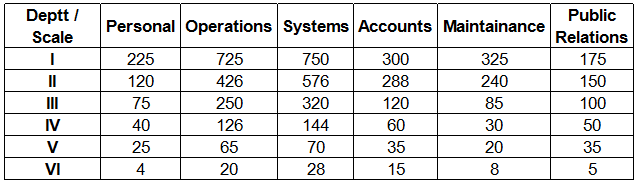0
250

# Data Analysis and Comparison Questions for XAT

Download Data Analysis and Comparison Questions for XAT PDF – XAT Data Analysis and Comparison questions PDF by Cracku. Practice XAT solved Data Analysis and Comparison Questions paper tests, and these are the practice question to have a firm grasp on the Data Analysis and Comparison topic in the XAT exam. Top 20 very Important Data Analysis and Comparison Questions for XAT based on asked questions in previous exam papers.  The XAT question papers contain actual questions asked with answers and solutions.

Instructions

Study the following graph carefully to answer these questions.
Per cent rise in population of two states from the previous year

Question 1: What was the effective percentage increase in population of state ‘A’ from 1999 to 2001 ?

a) 103

b) 85

c) 118

d) Cannot be determined

e) None of these

Solution:

Let the population of State A in 1999 = $100x$

=> Population in 2000 = $\frac{140}{100} \times 100x = 140x$

Population in 2001 = $\frac{145}{100} \times 140x = 203x$

$\therefore$ Effective % increase = $\frac{203x – 100x}{100x} \times 100$

= $\frac{103}{100} \times 100$ = 103%

Question 2: If the population of state A in 1998 was 67.5 lakhs, what was its population in 1996 ?

a) 50 lakhs

b) 42 lakhs

c) 45 lakhs

d) 40 lakhs

e) None of these

Solution:

Let the population of State A in 1996 = $100x$ lakhs

=> Population in 1997 = $\frac{125}{100} \times 100x = 125x$ lakhs

Population in 2008 = $\frac{135}{100} \times 125x = 168.75x$ lakhs

Acc to ques,

=> $168.75x = 67.5$

=> $x = 0.4$

$\therefore$ Population of State A in 1996 = 100 * 0.4 = 40 lakhs

Question 3: If population of state B in 1996 was 20 lakhs, what was the average population of the state in 1997 and 1998 ?

a) 22.5 lakhs

b) 25 lakhs

c) 27.6 lakhs

d) 24 lakhs

e) None of these

Solution:

Population of State B in 1996 = 20 lakhs

=> Population in 1997 = $\frac{120}{100} \times 20 = 24$ lakhs

Population in 1998 = $\frac{130}{100} \times 24 = 31.20$ lakhs

$\therefore$ Required Average

= $\frac{24 + 31.2}{2} = 27.6$ lakhs

Question 4: Total population of the two states in 1996 was 87.5 lakhs, what was the total population of the two states in 1998 ?

a) 107 lakhs

b) 22 lakhs

c) 105 lakhs

d) Cannot be determined

e) None of these

Solution:

We do not know the individual population of the two states. Thus, we cannot calculate the % increase in population of each states.

=> Ans – (D)

Question 5: If the ratio between the population of states A & B in year 2000 was 3 : 4 respectively, what was the respective ratio in 2001 ?

a) 7 : 9

b) 29 : 36

c) 81 : 116

d) Cannot be determined

e) None of these

Solution:

Let the population of State A in 2000 = $300x$

Population of State B in 2000 = $400x$

=> Population of State A in 2001 = $\frac{145}{100} \times 300x = 435x$

Population of State B in 2001 = $\frac{135}{100} \times 400x = 540x$

$\therefore$ Required ratio = $\frac{435x}{540x}$

= 29 : 36

Instructions

Study the following table to answer these questions. Number of Officers in various Departments of an Organization in different ScalesQuestion 6: What percentage of Scale IV officers are deployed in ‘Operations’ department ?

a) 26.5

b) 28

c) 28.5

d) 27

e) None of these

Solution:

Total number of scale IV employees = 40 + 126 + 144 + 60 + 30 + 50

= 450

$\therefore$ Required % = $\frac{126}{450} * 100$

= 28 %

Question 7: Out of the total number of employees in ‘Personnel’ department, approximately what per cent of employees are in scale II ?

a) 30

b) 28

c) 22

d) 20

e) 25

Solution:

Number of employees in Personnel department = 225 + 120 + 75 + 40 + 25 + 4

= 489

Number of employees in Scale II = 120

$\therefore$ Required % = $\frac{120}{489} * 100$

$\approx \frac{120}{490} * 100 = 24.48$

= 25% (approx.)

Question 8: In Public Relations department the number of employees in Scale II is less than that in Scale I by what percent? (rounded off to two digits after decimal).

a) 14.67

b) 16.67

c) 14.29

d) 16.27

e) None of these

Solution:

Number of employees in Public Relations department in :

Scale I = 175

Scale II = 150

Difference = 175 – 150 = 25

=> Required % = $\frac{25}{175} * 100$

= 14.29%

Question 9: Total number of employees in Scale VI is what per cent of the total number of employees in Scale-I ?

a) 2.8

b) 2.4

c) 3.6

d) 3.2

e) None of these

Solution:

Total number of employees in Scale I = 225 + 725 + 750 + 300 + 325 + 175 = 2500

Total number of employees in Scale VI = 4 + 20 + 28 + 15 + 8 + 5 = 80

=> Required % = $\frac{80}{2500} * 100$

= $\frac{80}{25}$ = 3.2 %

Question 10: What is the ratio between the total number of employees in Scale III and Scale IV respectively?

a) 19 : 9

b) 9 : 19

c) 17 : 9

d) 9 : 17

e) None of these

=> Required ratio = $\frac{950}{450}$